# Introduction to Machine Learning — Linear Regression

“Life is ten percent what you experience and ninety percent how you react to it.” — Charles R. Swindoll

Once you have gone through this tutorial, you will look at the above quote from a very different perspective.

Before we reason the above philosophical quote with mathematics, let us first face the toughest thing you’re going to encounter in this write-up — a Line. We know that a line connects a minimum of two points in order. But how does this simple piece of information help us master the science of prediction?

It’s simple!

In the case of linear regression, we use the concept of a line to help us make predictions. In case you’re wondering how that is done, this blog is just for you.

Let us see how a line works. A line is mathematically defined by the equation :

This equation contains ‘x’ which is the input point, ‘m’ which corresponds to the slope of said line and ‘c’ which is very commonly referred to as the ‘y-intercept’. The basic idea is that we provide this line an input ‘x’ and it throws back an output ‘y’ based on coefficients ‘m’ and ‘c’. In terms of machine learning, we term ‘x’ as feature variables (information about a particular data point) and ‘y’ as target variables (information that we want to predict based on ‘x’). And our aim is to find the relationship between ‘x’ and ‘y’ that will give us accurate predictions for our targets as possible.

So now that we have a basic understanding of how a line works mathematically, let us first understand why we call this model ‘linear regression’. Looking at the equation of a line, we see that since both the terms ‘x’ and ‘y’ exist in single dimensionality (i.e; in single powers) it aptly represents a linear relationship between them. From this we understand that this model works best when we have almost completely linear data, i.e; the features can be mapped to the target linearly.

Another interesting scenario is when data sets (like almost all real-life data sets) have multiple features and a single target. In the case of linearity being present, the kind of regression that we implement is called multiple regression. The mathematical equation of the model, in this case, remains quite similar to that of the former case, i.e;

At this point, let us look at the term — collinearity. Collinearity dictates that there exists some linear relationship between a particular feature variable and a target variable. So suppose we were to eliminate 2 out of 5 features from a data set and try to predict a target, we would be able to do so with a decent accuracy given the 2 features exhibit a good enough collinearity with the target data points. However, we also have to take care of the fact that the features shouldn’t exhibit collinearity amongst themselves. In such instances, the performance of our regression gets affected as a greater number of correlated/collinear features constitute noise in our data and skew the results of our regression.

As a rule of thumb, if the collinearity between the features and target is greater than 0.5, decent results can be expected from a linear regression model. Determining which variables may significantly impact the performance of our multiple regression model can be determined by various methods such as Backward Elimination, Forward Selection, and Bidirectional elimination. These topics will be covered in-depth in upcoming blogs

Until now, we have seen how we get outputs from a line. But in order to do so, we need values for ‘m’ and ‘c’ coefficients. Let us now see how for a given piece of data we can obtain optimum coefficients to find the best fitting line and use it to make predictions.

Before we go into the finer details let us get acquainted with let us go over some basic terminologies.

Error/Cost function — In most training processes we estimate how good an existing model is (in this case a line), by how well it fits the data given. In linear regression, we most commonly make use of a popular metric called mean squared error.

Gradient Descent — One of the most popular ways to determine the optimum coefficients for any model is gradient descent. Many of you must have heard of this term. For the ones who haven’t, let us break it down very simply. Gradient refers to the slope of a function and descent means moving down the determined slope. The idea behind this method is that we visualize our function as a mountain. To minimize this function, our goal is to reach the bottom of this peak. So our approach would be to take baby steps in the direction of reducing slope (gradient). Doing so iteratively brings us to the point where the reduction in slope becomes negligible and we reach our optimized point on the curve.

Let us now see how gradient descent helps us in determining the coefficients for our Linear Regression model.

Our original equation of a line :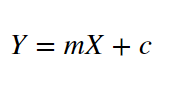With ‘m’ and ‘c’ as coefficients to be determined

Representation of our line with respect to data points (well-fitted line) :

Cost function based on the data and our line. Here yᵢ is the actual value and ȳᵢ is the predicted value.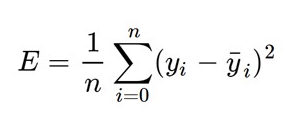Mean Squared Error between the test variables and predictions

Substituting the value of ȳᵢ :

Having derived our equation for the cost function, we are now ready to minimize it with respect to ‘m’ and ‘c’.

To calculate the partial derivative of E with respect to ‘m’ following the chain rule :

To calculate the partial derivative of E with respect to ‘c’ following the chain rule :

Having figured out the derivatives we are now ready to optimize our coefficients ‘m’ and ‘c’ such as :

Here ‘L’ is termed as something called the learning rate. This determines how quickly/slowly the descent happens. If the value of ‘L’ is too high descent happens quickly but there is a possibility of missing the lowest point in the function. However, if the value of ‘L’ is too low then the descent might take a very long while to reach the optimal point. The former guarantees a quicker but less accurate optimization however the latter guarantees a much slower but a higher accuracy and surety.

The key is to select a learning rate that falls in between the two cases. This is a part of hyper-parameter tuning that we will go deep into in another post.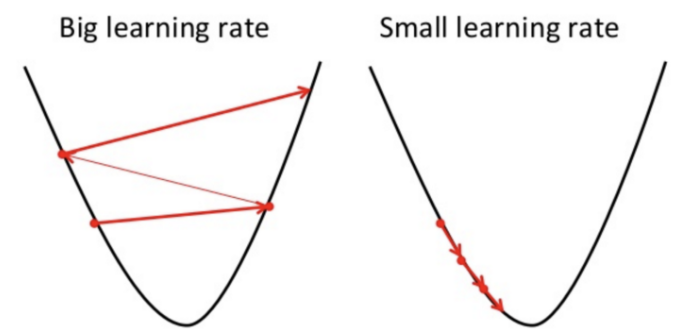Comparison between the two cases of learning rate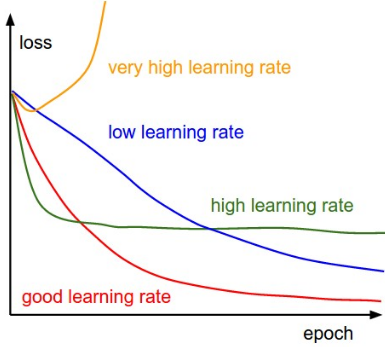Epoch Vs Loss w.r.t. the learning rate

The idea here is to perform the descent, i.e; perform the modifications for a certain number of time till the value of our error/cost function reduces to a value very close to 0. The lower the value, the better our function would have been optimized, and hence the better our coefficients ‘m’ and ‘c’ would be determined.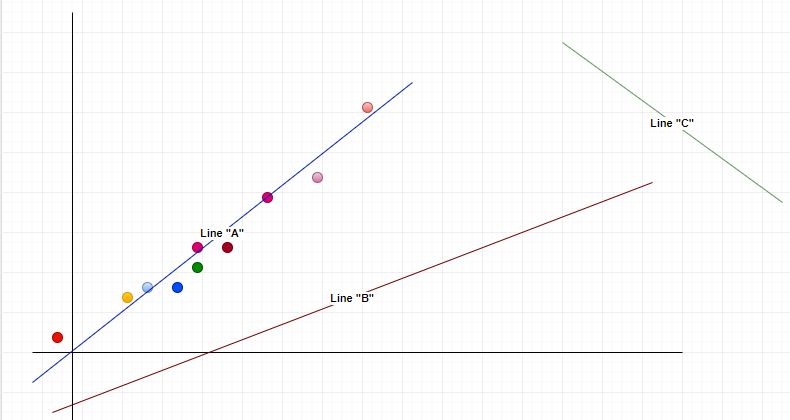Line “C” — line with initial parameters, Line “B” training after a few iterations, Line “A” — training after multiple iterations; “A” ends up fitting our data the best.

From the above plots, we understand how a model might be before and after it is trained on data respectively. To keep discussions simple we’ll just take a look at the terms underfitting and overfitting. Underfitting represents the scenario when the model is unable to satisfactorily understand the data at all and overfitting is when the model starts “memorizing” the training data. In both cases the model fails on test data, i.e; the data it has never seen before. Hence the best case scenario lies between the two (blue line in the above plot). To achieve the same we often divide the given data into training and validating samples. This is to make sure that our model is being trained well and isn’t over-fitting at the same time. Hence we constantly validate our model while training and this often brings out the best results with our test data.

Now that we’re at the end of the blog, does the quote at the beginning of this post make sense?

--

--